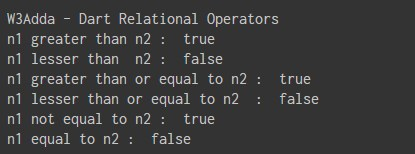# Dart Relational operators

In this tutorial you will learn about the Dart Relational operators and its application with practical example.

## Dart Relational Operators

Relational Operators are used evaluate a comparison between two operands. The result of a relational operation is a Boolean value that can only be true or false. Relational Operators are also referred as Comparison operators.

Let variable a holds 20 and variable b holds 10, then −

Dart Relational operators
Operator Description Example
`>` `greater than` ```a>b returns TRUE ```
`<` `Less than` ```a<b returns FALSE ```
`>=` `greater than or equal to` ```a>=b returns TRUE ```
`<=` `less than or equal to` ```a<=b returns FALSE ```
`==` `is equal to` ```a==b returns FALSE ```
`!=` `not equal to` `a!=b returns TRUE`

Example:-

When you run the above Dart program, you will see following output.

Output:-In this tutorial we have learn about the Dart Relational operators and its application with practical example. I hope you will like this tutorial.